# Dynamic Light Scattering (DLS) - An Introduction

Dynamic light scattering, sometimes known as quasi-elastic light scattering or photon correlation spectroscopy, is a method used for determining the particle size that typically in the sub-micron region.

## Brownian Motion

The DLS technique measures Brownian motion and correlates this to the particles’ size. The random movement of particles caused by the bombardment of the solvent molecules surrounding them is known as Brownian motion. DLS is generally associated with the measurement of particles suspended within a liquid.

If the particle is larger, the Brownian motion will be slower. The solvent molecules further “kick” the smaller particles, which move more quickly. For the DLS technique, an accurately known temperature is very important because viscosity knowledge is required (since the viscosity of a liquid is related to its temperature). Moreover, there must be a stable temperature, or else convection currents present in the sample will result in non-random movements that will affect the exact interpretation of size.

A property called the translational diffusion coefficient (often given the symbol, D) defines the velocity of the Brownian motion.

## The Hydrodynamic Diameter

A particle’s size is measured from the translational diffusion coefficient by applying the Stokes-Einstein equation: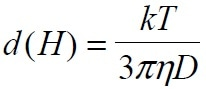where:

d(H) = hydrodynamic diameter

k = Boltzmann’s constant

T = absolute temperature

η = viscosity

D = translational diffusion coefficient

It is to be noted that the diameter measured in DLS is a value that refers to the way a particle diffuses within a fluid, and therefore it is called a hydrodynamic diameter. The diameter acquired through this method is the diameter of a sphere which possesses the same translational diffusion coefficient as that of the particle.

Not only does the translational diffusion coefficient depend on the size of the particle “core”, but it also relies on any surface structure, as well as the type and concentration of ions in the medium. Factors affecting the particles’ diffusion speed are discussed in the following sections.

### Ionic Strength of Medium

By altering the thickness of the electric double layer known as the Debye length (K-1), the ions in the medium and the total ionic concentration can influence the diffusion rate of particles. Therefore, a low conductivity medium will create an extended double layer of ions around the particle; this reduces the diffusion speed and results in a larger and rather obvious hydrodynamic diameter. However, if higher conductivity media are used, they will suppress the electrical double layer and the calculated hydrodynamic diameter.

Usually, the performance of a DLS instrument is checked by measuring an appropriate polystyrene latex standard. If the standard has to be diluted before measurement, then dilution should be made in an appropriate medium. According to the International Standard on DLS (ISO13321 Part 8 1996), dilution of any polystyrene standard has to be made in 10 mM NaCl. This salt concentration will suppress the electrical double layer and make sure that the hydrodynamic diameter reported remains the same as the hydrodynamic diameter on the certified or the predicted diameter.

### Surface Structure

If there is any change in a particle surface that impacts the diffusion speed, it will correspondingly change the apparent size of the particle. The diffusion speed will reduce further if an adsorbed polymer layer projects out into the medium than if the polymer lies flat on the surface. The nature of the polymer and the surface, as well as the medium’s ionic concentration, can influence the polymer conformation, which will subsequently change the obvious size by several nanometers.

### Non-Spherical Particles

All particle-sizing methods are limited when it comes to describing the size of non-spherical particles. The only object is the sphere, whose size can be accurately described by a single figure.

Different methods are sensitive to different properties of the particle, for example, scattering intensity, projected area, and density will generally produce different size distributions and mean sizes for any given sample. In fact, even the size in a microscope image will be dependent on parameters set such as edge contrast and so on. It must be understood that none of these results are innately “correct.”

In the case of a non-spherical particle, its hydrodynamic diameter is the diameter of a sphere that possesses the same translational diffusion speed as that of the particle. The hydrodynamic size will change if the shape of a particle changes in a way that influences the diffusion rate. For instance, slight variations in the length of a rod-shaped particle will directly impact the size, while variations in the rod’s diameter, which will barely influence the diffusion rate, will be hard to detect.

The conformation of macromolecules and proteins often depend on the precise nature of the dispersing medium. Since conformational changes will generally influence the diffusion speed, DLS is a highly sensitive method for detecting these changes.

## Light Scattering Theories

### Rayleigh Scattering

If the particles are small in comparison to the wavelength of the laser used (usually less than d = λ/10 or approximately 60 nm for a He-Ne laser), the scattering from a particle illuminated by a vertically polarized laser will be in essence isotropic, that is, equal in all directions.

The Rayleigh approximation shows that I ∝ d6 and also that I ∝ 1/λ4, where I is the intensity of light scattered, d is the diameter of a particle, and λ is the laser wavelength. The d6 term shows that a 50 nm particle will scatter one million or 106 times as much light as a 5 nm particle. Therefore, there is a risk that the light emitted from the larger particles will swamp the scattered light emitted from the smaller particles. This d6 factor also means that it would be hard to measure, for example, a mixture of 10 nm and 1000 nm particles with DLS, because the contribution to the total light scattered by the small particles will be very small. The inverse relationship to λ4 means that as the wavelength of the laser used decreases, a higher scattering intensity is achieved.

### Mie Theory

When the particles’ size becomes almost equivalent to the wavelength of the illuminating light, a complex function of minima and maxima with regards to angle can be seen. Shown in Figure 1 is the theoretical plot of the log of the relative scattering intensity against the particle size at 173° angle (the detection angle of the Zetasizer Nano ZS and Nano S in aqueous media) and 90° angle (the detection angle of the Nano ZS90 and Nano S90) assuming an imaginary refractive index of 0.001, real refractive index of 1.59, and laser wavelength of 633 nm.

Mie theory happens to be the only theory that correctly explains the minima and maxima in the plot of intensity with angle and provides the right answer over all angles, sizes, and wavelengths. This theory is employed in the Nano software for changing the intensity distribution into volume.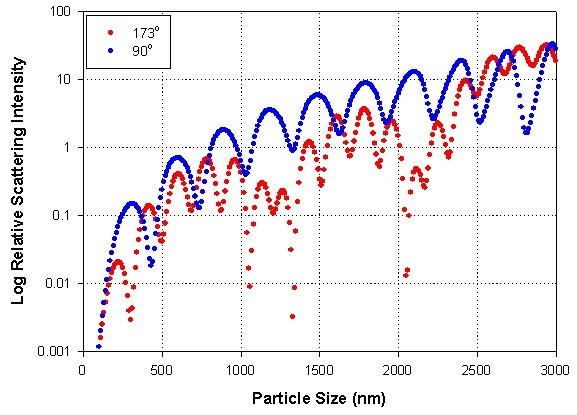Figure 1. Theoretical plot of the log of the relative intensity of scattering versus particle size at angles of 173° (the detection angle of the Nano S, and Nano ZS in aqueous media) and 90° (the detection angle of the Nano S90 and Nano ZS90) assuming a laser beam at a wavelength of 633 nm, real refractive index of 1.59, and an imaginary refractive index of 0.001.

## How DLS Works

The speed at which the particles are diffusing as a result of Brownian motion is measured in DLS. This is achieved by determining the rate at which the intensity of the scattered light varies when detected with an appropriate optical arrangement. However, it is not known how these fluctuations in the intensity of scattered light arise.

Consider an example where a cuvette, containing stationary particles, is illuminated by a laser and a frosted glass screen is used for viewing the sample cell. A standard speckle pattern would be observed, as shown in Figure 2.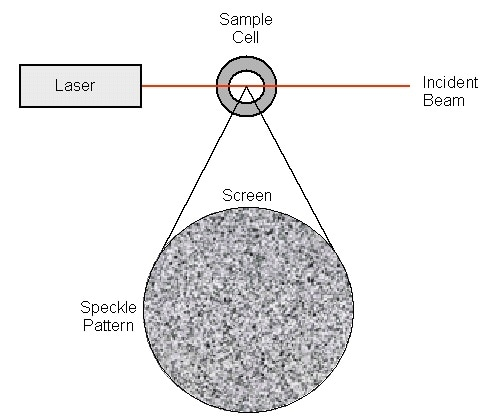Figure 2. Schematic representation of a speckle pattern.

Since the entire system is stationary, the speckle pattern will be stationary both in terms of speckle position and size. The dark spaces are areas where the phase additions of the scattered light are equally destructive and cancel each other out (see Figure 3A). Within the speckle pattern, the bright blobs of light are the areas where the light scattered from the particles arrives with the same equal phase and impedes constructively to create a bright patch (see Figure 3B).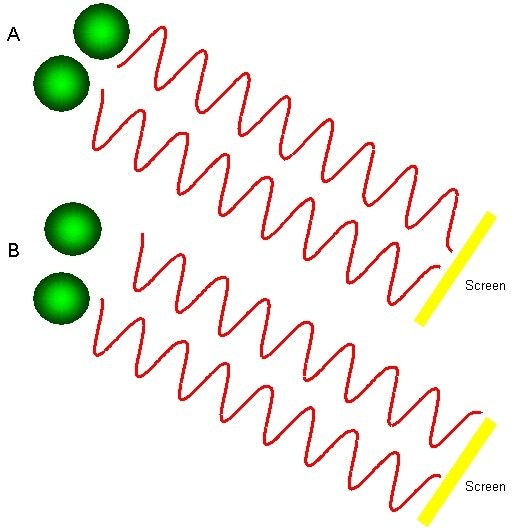Figure 3. The observed signal depends on the phase addition of the scattered light falling on the detector. In example A, two beams interfere and “cancel each other out,” resulting in a decreased intensity detected. In example B, two beams interfere and “enhance each other,” resulting in an increased intensity detected.

For a system of particles experiencing Brownian motion, a speckle pattern is seen where the position of each speckle is observed to be in constant motion, because the moving particles’ phase addition is continuously evolving and forming new patterns. The size of the particles decide the speed at which these intensity fluctuations take place. Typical intensity fluctuations arising from a dispersion of both small and large particles are schematically shown in Figure 4. It is the small particles that cause the intensity to fluctuate more quickly than the large ones.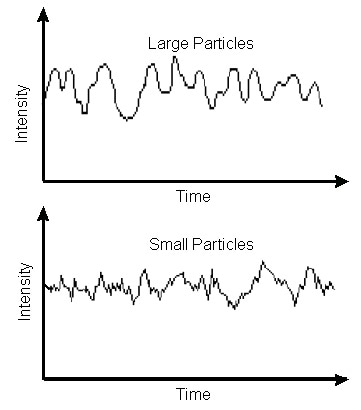Figure 4. Typical intensity fluctuations for large and small particles.

While you can calculate the spectrum of frequencies contained in the intensity fluctuations arising from the Brownian motion of particles, it is inefficient to do so. Using a device known as a digital autocorrelator is the best way to do this.

## How a Correlator Works

A correlator is essentially a signal comparator designed for measuring the degree of resemblance between two signals, or a single signal with itself at variable time intervals.

If the signal intensity is compared with itself at a specific point in time and a time much later, then it is apparent for a randomly fluctuating signal that the intensities are not going to be associated in any way, that is, no correlation exists between the two signals as shown in Figure 5. Knowledge of the initial signal intensity will make it impossible to predict the signal intensity at time t = infinity. This is true of any random process, for example, diffusion.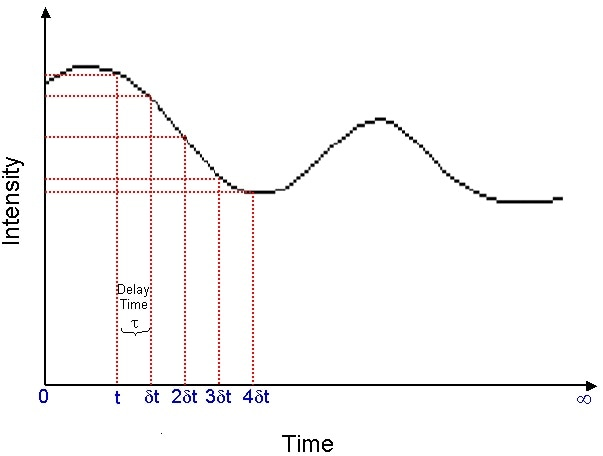Figure 5. Schematic showing the fluctuation in the intensity of scattered light as a function of time.

Conversely, upon comparing the intensity of the signal at time = t to the intensity a very small time later (t + δt), a strong relationship or correlation will exist between the intensities of two signals. The two signals are well or strongly correlated.

If the signal, obtained from a random process like Brownian motion, at t is compared to the signal at t + 2δt, a reasonable correlation or comparison will still be there between the two signals; however, it will not be as good as the comparison at t and t + δt. This correlation reduces with time. Usually, the period of time δt is extremely small, perhaps microseconds or nanoseconds, and is known as the sample time of the correlator. t = ∞ possibly of the order of a millisecond or tens of milliseconds.

If the intensity of the signal at t is compared with itself, then there is a seamless correlation as the signals are identical. No correlation is indicated by zero (0.00) and perfect correlation is indicated by unity (1.00).

Upon comparing the signals at t + 2δt, t + 3δt, t + 4δt, and so on with the signal at t, the correlation of a signal arriving from a random source will reduce with time until at some time, effectively t = ∞, no correlation will be there.

If there are large particles, the signal will change gradually and the correlation will continue for a long time as shown in Figure 6. However, the correlation will reduce more rapidly if the particles are small (see Figure 7).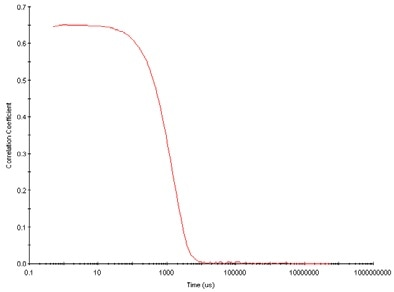Figure 6. Typical correlogram from a sample containing large particles in which the correlation of the signal takes a long time to decay.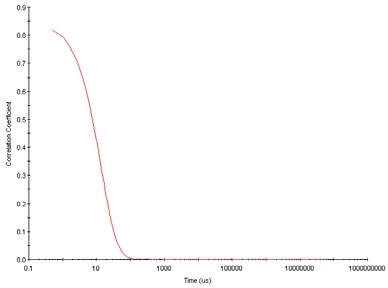Figure 7. Typical correlogram from a sample containing small particles in which the correlation of the signal decays more rapidly.

Observing the correlogram from a measurement can provide plenty of information regarding the sample. The time at which the correlation begins to considerably decay indicates the mean size of the sample. If the line is steeper, the sample will be more monodispersed. However, if the decay becomes more extended, the sample polydispersity will be greater.

### The Correlation Function

It has been observed that particles in a dispersion are in a continuous, random Brownian motion and it is this which causes the intensity of scattered light to vary as a function of time. The correlator employed in a PCS instrument will build the correlation function G(τ) of the scattered intensity:

G(τ) = <I(t).I(t+τ)>

where τ is the correlator’s time difference (the sample time).

For a huge number of monodisperse particles in Brownian motion, the correlation function (which is given the symbol [G]) is an exponential decaying function of the correlator time delay τ:

G(τ) = A[1 + B exp(-2Γ τ)]

where A is the baseline of the correlation function, and B is the intercept of the correlation function.

Γ = Dq2

where D is the translational diffusion coefficient

q = (4 π n / λo) sin (θ/2)

where n is the refractive index of dispersant, λo is the wavelength of the laser, and θ is the scattering angle.

The following equation can be written for polydisperse samples:

G(τ) = A[1 + B g1 (τ)2]

where g1(τ) is the sum of all the exponential decays contained in the correlation function.

### Obtaining Size Information from the Correlation Function

Various algorithms are used to obtain the size from the correlation function. Two approaches are available that can be taken: (1) one method is to fit a single exponential to the correlation function to achieve the mean size (z-average diameter) and take an estimate of the width of the distribution (polydispersity index) (this is known as the Cumulants analysis and is defined in ISO13321 Part 8), or (2) the other method is to fit a multiple exponential to the correlation function to achieve the distribution of particle sizes (for example, non-negative least squares (NNLS) or CONTIN.

The size distribution, thus acquired, is a plot of the relative intensity of light scattered by particles in different size classes and is hence called an intensity size distribution.

If the distribution by intensity turns out to be a single and relatively smooth peak, then there is not much point in making the conversion to a volume distribution through the Mie theory. In case the optical parameters are correct, this will merely give a peak of slightly different shape. Conversely, if the plot demonstrates a considerable tail, or more than a single peak, then Mie theory can change the intensity distribution to a volume distribution by leveraging the input parameter of sample refractive index. This will subsequently offer a more realistic view of the significance of the tail or the presence of the second peak. In general terms, it will be observed that:

d(intensity) > d(volume) > d(number)

One easy way to describe the variation between volume, intensity, and number distributions is to account for two populations of spherical particles of diameter — 50 nm and 5 nm — present in equal numbers, as illustrated in Figure 8.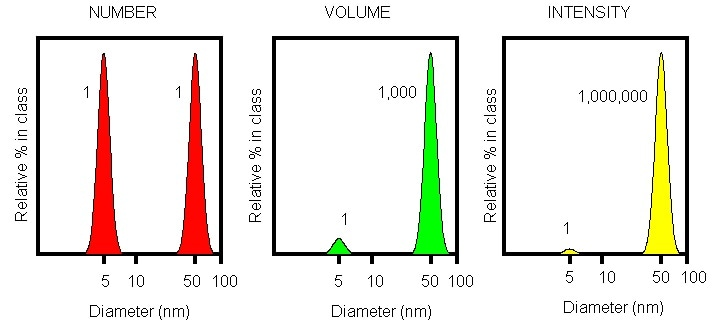Figure 8. Number, volume, and intensity distributions of a bimodal mixture of 5 and 50 nm lattices present in equal numbers.

Upon plotting a number distribution of these two particle populations, a plot containing two peaks (positioned at 5 and 50 nm) of a ratio of 1:1 will be achieved. If this number distribution was changed into volume, the two peaks would then change to a ratio of 1:1000 (because a sphere’s volume is equal to 4/3π(d/2)3). If this is further changed into an intensity distribution, a ratio of 1:1000000 between the two peaks will be achieved (this is because the intensity of scattering is proportional to d6 (from Rayleigh’s approximation)). It should be remembered that in DLS, the distribution achieved from a measurement is based on intensity.

## Optical Configuration of a Dynamic Light Scattering Instrument

In a typical dynamic light scattering system, six key components are present. First, a light source is provided by a laser ➀ to illuminate the sample contained in a cell ➁. For dilute concentrations, while a large part of the laser beam travels through the sample, some of it is scattered by the particles inside the sample at all angles. A detector ➂ is used for measuring the scattered light. The detector position in the Zetasizer Nano series will be at either 90° or 173°, based on the specific model.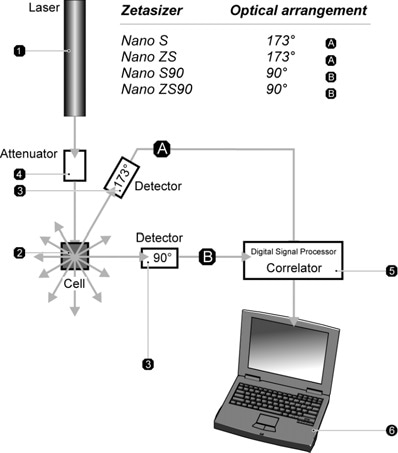Figure 9. Optical configurations of the Zetasizer Nano series for dynamic light scattering measurements.

It is important that the intensity of scattered light lies within a particular range so that the detector can effectively measure it. The detector will become saturated if an excess amount of light is detected. In order to resolve this, an attenuator ➃ is used to minimize the laser source intensity and thus reduce the scattering intensity. For samples of low concentration or samples that do not scatter too much light, for example, very tiny particles, the amount of scattered light should be increased. In this case, the attenuator will enable more laser light to pass through to the sample.

For samples at higher concentration or samples that scatter more light, for example, large particles, the intensity of scattered light should be decreased. The Nano software automatically determines the appropriate position of the attenuator, covering a transmission range of 100% to 0.0003%.

The detector’s scattering intensity signal is passed to a digital processing board known as a correlator ➄. It is the correlator that compares the intensity of scattering at successive time intervals in order to derive the rate at which the intensity fluctuates. This correlator data is subsequently passed to a computer ➅, where the Nano software will examine the data and derive information related to size.

## Unique Features of the Zetasizer Nano

### Non-Invasive Backscatter Detection (NIBS)

The Nano ZS and Nano S instruments are designed to detect the scattering information at 173°. This is called backscatter detection. Moreover, the optics do not come into contact with the sample and so the detection optics are said to be non-invasive.

The application of non-invasive backscatter detection provides a number of advantages:

• There is no need for the laser to travel through the whole sample. This reduces an effect referred to as multiple scattering, where other particles scatter light from a signal particle. When the light travels through the sample’s shorter path length, higher concentrations of the sample can be subsequently determined.
• Dust particles and other contaminants inside the dispersant are usually large when compared to the size of the sample. Large particles primarily scatter in the forward direction. Hence, the effects of dust are considerably reduced by using backscatter detection.

### Variable Measurement Position for Sizing

It is possible to change the measurement position inside the cuvette of the Nano S and Nano ZS instruments. By moving the focusing lens, this measurement position is altered and is automatically determined by the Nano software (see Figure 10).

In the case of small particles, or low concentrations of samples, it is advantageous to increase the amount of scattering from the sample. The laser will cause “flare”, as the laser passes through the cuvette wall and into the dispersant. This flare can possibly swamp the signal from the scattering particles. This effect can be removed by moving the measurement point away from the wall of the cuvette and towards the middle of the cuvette (see Figure 10a).

Relatively more amount of light is scattered by large particles or samples at high concentrations. In such a case, measuring closer to the wall of the cuvette will lower the effect of multiple scattering by reducing the path length over which the scattered light has to pass (see Figure 10b). A combination of the intercept of the correlation function and the intensity of the light scattered enables automatic determination of the measurement position.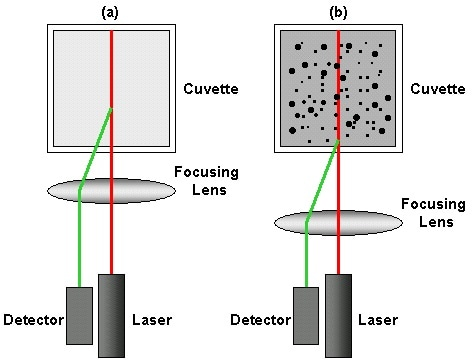Figure 10. Schematic diagram showing the measurement position for (a) small, weakly scattering samples and for (b) concentrated, opaque samples. The change in measurement position is achieved by moving the focusing lens accordingly.

1. International Standard ISO13321 Methods for Determination of Particle Size Distribution Part 8: Photon Correlation Spectroscopy, International Organization for Standardization (ISO) 1996.
2. Dahneke, B.E. (ed) Measurement of Suspended Particles by Quasi-elastic Light Scattering, Wiley, 1983.
3. Pecora, R. Dynamic Light Scattering: Applications of Photon Correlation Spectroscopy, Plenum Press, 1985.
4. Washington, C. Particle Size Analysis in Pharmaceutics and Other Industries: Theory And Practice, Ellis Horwood, England, 1992.
5. Johnson, C.S. Jr. and Gabriel, D.A. Laser Light Scattering, Dover Publications, Inc., New York 1981.This information has been sourced, reviewed and adapted from materials provided by Micromeritics Instrument Corporation.

## Citations

• APA

Micromeritics Instrument Corporation. (2019, December 09). Dynamic Light Scattering (DLS) - An Introduction. AZoM. Retrieved on October 22, 2020 from https://www.azom.com/article.aspx?ArticleID=16774.

• MLA

Micromeritics Instrument Corporation. "Dynamic Light Scattering (DLS) - An Introduction". AZoM. 22 October 2020. <https://www.azom.com/article.aspx?ArticleID=16774>.

• Chicago

Micromeritics Instrument Corporation. "Dynamic Light Scattering (DLS) - An Introduction". AZoM. https://www.azom.com/article.aspx?ArticleID=16774. (accessed October 22, 2020).

• Harvard

Micromeritics Instrument Corporation. 2019. Dynamic Light Scattering (DLS) - An Introduction. AZoM, viewed 22 October 2020, https://www.azom.com/article.aspx?ArticleID=16774.